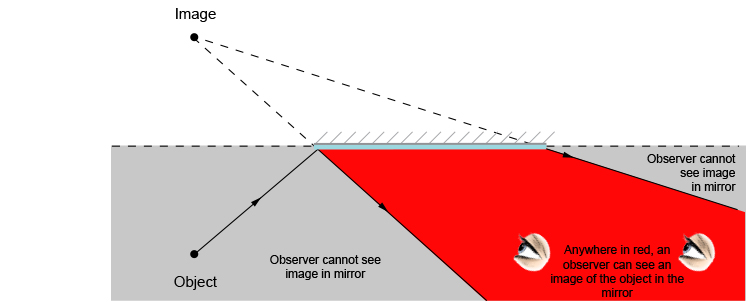# Where can an observer see a reflected image?

If in an exam you were asked to find the area where an observer can see the image in a mirror, you would do so as follows: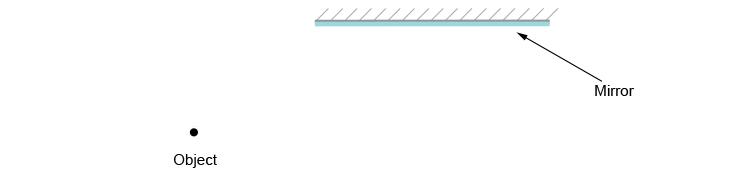You need to apply the laws of reflection and initially draw an image on the other side of the mirror perpendicular (at 90°) to the mirror.

Behind the mirror
Equal distance
Same size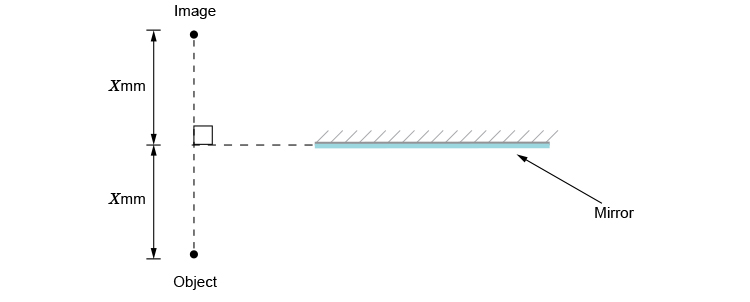We end up with a diagram as follows: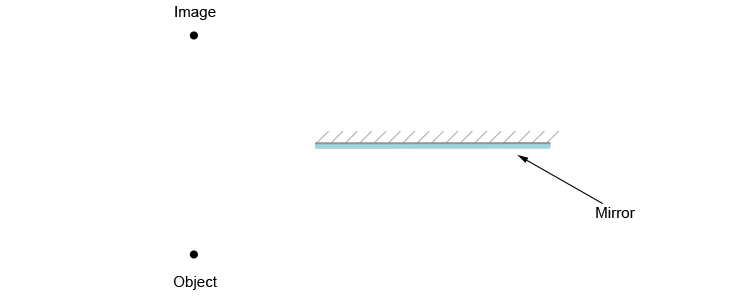The second stage is to draw rays from the object to the edges of the mirror and reflect off the edges, either

1. So that the angle of incidence equals the angle of reflection
Or
2. Draw from the image to the points on the edges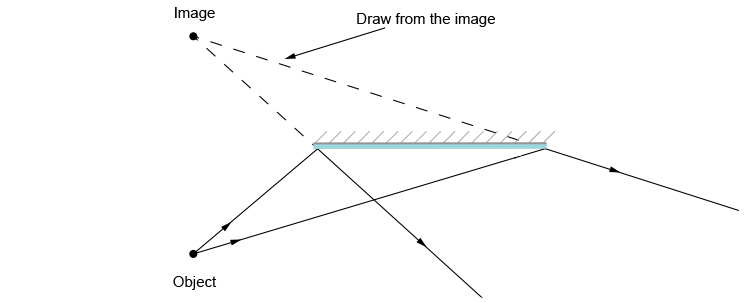Between these two reflected lines any observer will be able to see a reflected image.